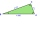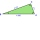# Right triangle calculator - result

Please enter two properties of the right triangle

Use symbols: a, b, c, A, B, h, T, p, r, R

You have entered cathetus a and cathetus b.

### Right scalene triangle.

Sides: a = 15   b = 36   c = 39

Area: T = 270
Perimeter: p = 90
Semiperimeter: s = 45

Angle ∠ A = α = 22.6219864948° = 22°37'11″ = 0.39547911197 rad
Angle ∠ B = β = 67.3880135052° = 67°22'49″ = 1.17660052071 rad
Angle ∠ C = γ = 90° = 1.57107963268 rad

Height: ha = 36
Height: hb = 15
Height: hc = 13.84661538462

Median: ma = 36.77329520164
Median: mb = 23.43107490277
Median: mc = 19.5

Vertex coordinates: A[39; 0] B[0; 0] C[5.76992307692; 13.84661538462]
Centroid: CG[14.92330769231; 4.61553846154]
Coordinates of the circumscribed circle: U[19.5; -0]
Coordinates of the inscribed circle: I[9; 6]

Exterior (or external, outer) angles of the triangle:
∠ A' = α' = 157.3880135052° = 157°22'49″ = 0.39547911197 rad
∠ B' = β' = 112.6219864948° = 112°37'11″ = 1.17660052071 rad
∠ C' = γ' = 90° = 1.57107963268 rad

# How did we calculate this triangle?

The calculation of the triangle progress in two phases. The first phase is such that we try to calculate all three sides of the triangle from the input parameters. The first phase is different for the different triangles query entered. The second phase is the calculation of other characteristics of the triangle, such as angles, area, perimeter, heights, the center of gravity, circle radii, etc. Some input data also results in two to three correct triangle solutions (e.g., if the specified triangle area and two sides - typically resulting in both acute and obtuse) triangle).

### 2. From cathetus a and cathetus b we calculate hypotenuse c - Pythagorean theorem:

Now we know the lengths of all three sides of the triangle, and the triangle is uniquely determined. Next, we calculate another its characteristics - same procedure as calculation of the triangle from the known three sides SSS.

### 4. Semiperimeter of the triangle

The semiperimeter of the triangle is half its perimeter. The semiperimeter frequently appears in formulas for triangles that it is given a separate name. By the triangle inequality, the longest side length of a triangle is less than the semiperimeter.

### 7. Calculation of the inner angles of the triangle - basic use of sine function

An incircle of a triangle is a circle which is tangent to each side. An incircle center is called incenter and has a radius named inradius. All triangles have an incenter, and it always lies inside the triangle. The incenter is the intersection of the three angle bisectors. The product of the inradius and semiperimeter (half the perimeter) of a triangle is its area.

The circumcircle of a triangle is a circle that passes through all of the triangle's vertices, and the circumradius of a triangle is the radius of the triangle's circumcircle. Circumcenter (center of circumcircle) is the point where the perpendicular bisectors of a triangle intersect.

$R=\frac{c}{2}=\frac{39}{2}=19.5$

### 10. Calculation of medians

A median of a triangle is a line segment joining a vertex to the midpoint of the opposite side. Every triangle has three medians, and they all intersect each other at the triangle's centroid. The centroid divides each median into parts in the ratio 2:1, with the centroid being twice as close to the midpoint of a side as it is to the opposite vertex. We use Apollonius's theorem to calculate the length of a median from the lengths of its side.

The right triangle calculators compute angles, sides (adjacent, opposite, hypotenuse) and area of any right-angled triangle and use it in the real world. Two independent properties entirely determine any right-angled triangle. The calculator provides a step-by-step explanation for each calculation.

A right triangle is a kind of triangle that has one angle that measures C=90°. In a Right triangle, the side c that is opposite of the C=90° angle, is the longest side of the triangle and is called the hypotenuse. The variables a, b are the lengths of the shorter sides, also called legs or arms. Variables for angles are A, B, or α (alpha) and β (beta). Variable h refers to the altitude(height) of the triangle, which is the length from the vertex C to the hypotenuse of the triangle.

Examples for right triangle calculation:

## A right triangle in word problems in mathematics:

• Triangle P2Can triangle have two right angles?
• Vector 7Given vector OA(12,16) and vector OB(4,1). Find vector AB and vector |A|.
• Height 2Calculate the height of the equilateral triangle with side 38.
• Area of RT 2Calculate the area of right triangle whose legs have a length 5.8 cm and 5.8 cm.
• Cable carCable car rises at an angle 45° and connects the upper and lower station with an altitude difference of 744 m. How long is "endless" tow rope?
• ABS CNCalculate the absolute value of complex number -15-29i.
• ChaunceyChauncey is building a storage bench for his son’s playroom. The storage bench will fit into the corner and against two walls to form a triangle. Chanuncy wants to buy a triangular shaped cover for the bench. If the storage bench is 2 1/2 ft. Along one wa
• CablewayCableway has a length of 1800 m. The horizontal distance between the upper and lower cable car station is 1600 m. Calculate how much meters altitude is higher upper station than the base station.
• Euclid2In right triangle ABC with right angle at C is given side a=27 and height v=12. Calculate the perimeter of the triangle.
• Trapezoid - RRFind the area of the right angled trapezoid ABCD with the right angle at the A vertex; a = 3 dm b = 5 dm c = 6 dm d = 4 dmThe ladder has a length 3.5 meters. He is leaning against the wall so that his bottom end is 2 meters away from the wall. Determine the height of the ladder.
• Four ropesTV transmitter is anchored at a height of 44 meters by four ropes. Each rope is attached at a distance of 55 meters from the heel of the TV transmitter. Calculate how many meters of rope were used in the construction of the transmitter. At each attachment
• BisectorsAs shown, in △ ABC, ∠C = 90°, AD bisects ∠BAC, DE⊥AB to E, BE = 2, BC = 6. Find the perimeter of triangle △ BDE.
• RT triangle and heightCalculate the remaining sides of the right triangle if we know side b = 4 cm long and height to side c h = 2.4 cm.
• Spruce heightHow tall was spruce that was cut at an altitude of 8m above the ground and the top landed at a distance of 15m from the heel of the tree?